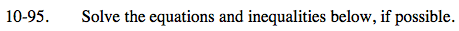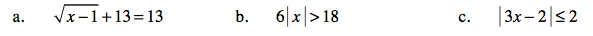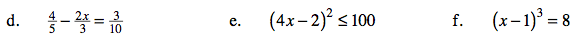### Home > AC > Chapter 10 > Lesson 10.2.5 > Problem10-95

10-95.Subtract 13 from both sides.

$\sqrt{x-1}=0$

Square both sides.
x − 1 = 0

x = 1

Divide both sides by 6.

|x| > 3

Find the boundary point(s).
x = −3 or x = 3

Test x = −4, x = 0, and x = 4.

6|− 4| > 18 24 > 18 True
6|0| > 18 0 > 18 False
6|4| > 18 24 > 18 True

x < −3 or x > 3

3x − 2 = 2 or 3x − 2 = −2

3x = 4 or 3x = 0

$x=\frac{4}{3} \text{ or } x=0$

$0\leq x \leq \frac{4}{3}$Multiply the equation by 30.

$\frac{120}{5}-\frac{60x}{3}=\frac{90}{10}$

24 − 20x = 9

Subtract 24 from both sides.
−20x = −15

Divide by −20 on both sides and simplify.

$x=\frac{-15}{-20}$

Follow the steps shown in parts (b) and (c).

See the hints and steps provided in the previous parts of this problem.